Schottky Barrier Formation

Donghyun Kim March 22, 2007

(Submitted as coursework for AP272, Stanford University, Winter 2007)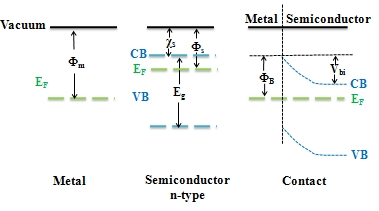Fig. 1: Schottky barrier at the contact between the metal and the semiconductor with the different electron affinities.

Introduction

The Schottky barrier is the rectifying barrier for electrical conduction across heterojunction , either the metal-semiconductor junction or semiconductor-semiconductor junction, and, therefore, is of vital importance to the successful operation of any semiconductor device. A metal-semiconductor junction that does not rectify current is called an Ohmic contact.

Electrical Behavior of Schottky barrier can be referred to as the thermionic emission current  where the majority carriers move over the potential barrier. Capacitance-voltage (C-V) method  is a convenient and common method for evaluating the Schottky barrier height of a Schottky contact.

The magnitude of the Schottky barrier height  reflects the mismatch in the energy position of the majority carrier band edge of the semiconductor and the metal Fermi level across the metal semiconductor interface and the surface states of the semiconductor. The origin of surface states in metal-semiconductor junction was disputed for decades. The defect model and the Metal Induced Gap States were suggested. [4, 5, 6, 7]

Electrical Behavior of Schottky Barrier

Current-Voltage Relationship

The current transport in a metal-semiconductor junction is due mainly to majority carriers as opposed to minority carriers in a pn junction. The basic process in the rectifying contact with an n-type semiconductor is by transport of electrons over the potential barrier, which can be described by the thermionic emission theory.

The net current density in the metal to semiconductor junction can be written as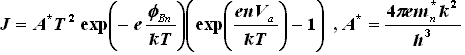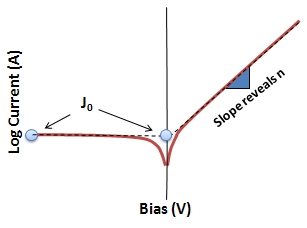Fig. 2: I-V relation in the rectifying Schottky diode.

where Va = applied bias, n=realization factor (fitting factor) and φBn= Schottky barrier height.

Several effects will alter the actual Schottky barrier MS junction current from the theoretical value given by the equation above. Tunneling current is important when large doping concentration in the semiconductor region is considered. Schottky effect or image force induced lowering of the barrier height should be included as well when there is large doping concentration.

C-V Measurement of Schottky Barrier Height

Capacitance-voltage (C-V) method is a common method for evaluating the Schottky barrier height of a Schottky contact. For the uniformly doped semiconductor, we have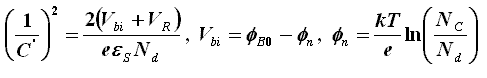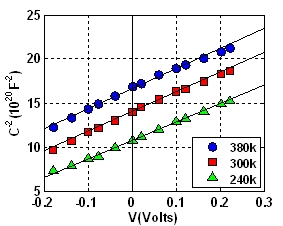Fig. 3: Temperature variation of C2 vs V characteristics at 1 MHz for the Au-pInP diode. 

The built-in potential Vbi can be extrapolated from the intercept in the voltage axis in the straight line of 1/C2 versus V plot. Once we have Vbi then the Schottky Barrier Height can be extracted by plugging Vbi in to the above equations.

Schottky Barrier Formation

Fermi Level Pinning

The question of how the Schottky barrier height (SBH) is determined is not well answered for long time. The first-order theory of the formation of a Schottky barrier is the view attributed to W. Schottky and Sir Mott. The Schottky-Mott theory proposes that the Schottky barrier height depends sensitively on the work function of the metal (SBH=Workfunction of metal-Fermi level of Semiconductor). However, this prediction has received little support from experiment. The Schottky barrier heights measured in actual experiments often showed some dependence on the preparation of the MS interface, which indicates that the Schottky barrier height depends more than just the work function of the metal. Despite some scatters in the experimental data, by and large, metals with larger work functions have been found to have systematically higher Schottky barrier heights than those with lower work functions. But the actual dependence observed is much weaker than that predicted by the Schottky-Mott theory. A term, "Fermi level pinning", has often been used to describe the insensitivity of the experimental Schottky barrier height to the metal work function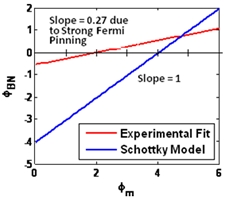Fig. 4:Si Schottky barrier height and the prediction by Schottky model (SBH = workfunction of metal - Fermi level of Si.). Measured Schottky barrier height shows strong Fermi level pinning. 

Quantum Dipole Caused by Interface States

Cowley and Sze  explained the discrepancy between Schottky model and experimentally measured Schottky barrier height by introducing interface state. The interface states are of the order of atomic dimensions and located between the metal and the semiconductor. They assumed this surface states density is a property of the semiconductor and is independent of the metal. The interface states create strong quantum dipoles between the metal and the semiconductor. These quantum dipoles create very large electric field at the junction and can alter the significant amount of potential within the range of atomic length.

Within the above assumptions, the barrier height for n-type semiconductor-metal contacts is found to be a linear combination of the metal work function φm and a quantity φo which is defined as the energy below which the surface states must be filled for charge neutrality at the semiconductor surface. The φo is measured from the edge of the valence band. For constant surface state density and low semiconductor doping concentration, the theoretical expression obtained is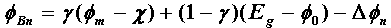where χ and Eg are electron affinity and the band gap of the semiconductor, respectively, Δφn is the image charge barrier lowering, and γ is a weighting factor which depends mainly on the surface state density and the thickness of the interfacial layer. According to Cowley and Sze's model the surface state density is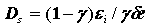where δ is a interface layer thickness and usually it is assumed to be less than lattice constant.

The special cases of this equation are of interest. First, if γ ≈ 0 then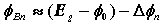In this case the Fermi level at the interface is pinned by the surface states at the value φo above the valence band. The barrier height is independent of the metal work function, and is determined entirely by the doping and surface properties of the semiconductor. Second, if γ ≈ 1, then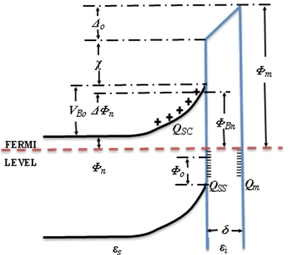Fig. 5: Si Schottky barrier height and the prediction by Schottky model (SBH = workfunction of metal – Fermi level of Si.). Measured Schottky barrier height shows strong Fermi level pinning.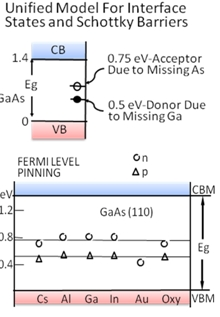Fig. 6: Unified Defect Model by Spicer.  Left figure shows the defect levels of several cleaved semiconductor and Right figure shows the Fermi pinning levels for thin metal layer deposited on the Semiconductors.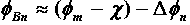This leads to the Schottky model where surface effects are neglected. This model enables us to predict the surface state density by estimating γ factor from experimental data. From figure 4, the surface state density for the Si is obtained as only 0.02 state/atom eV and it is enough to exhibit Fermi pinning at the metal-semiconductor junction.  To explain the origin of the surface states in metal semiconductor junctions, there had been two major attempts. First is the Defect model by Spicer and the second is the Metal Induced Gap State originally brought by Heine and was backed by more rigorous pseudopotential calculations by Louie, Chelikowsky and Cohen.

Spicer's Defect Model

Spicer  claimed that the surface states created by dangling bond of covalent semiconductor is the major reason for Fermi level pinning observed at the MS junction. He deposited only few monolayers of metals on the Semiconductors and measured the fermi level of the film. Experimentally he showed the evidence for defect pinning of Fermi level (Fig 6.) on surfaces with submonolayer metal coverage. However the results of these experiments were inconsistent with bulk barrier height measurements. In his lab, few monolayers of metal were not successfully deposited uniformly, rather those are bunched up. And some of the metals he used do not show metallic behavior at the monolayer thickness. Furthermore, more rigorous calculations done by Louie et al. showed that the intrinsic surface states of semiconductor or so called defects were removed by interaction with metal atoms and new surface states appeared due to the presence of the metal.

Metal Induced Gap States (MIGS)

The Metal Induced Gap State originally was introduced by Heine  and was backed by more rigorous pseudopotential calculations by Louie, Chelikowsky and Cohen .

In 1965 Heine  pointed out that localized surface states cannot exist in the semiconductor when it is contacting the metal, but resonance surface states which are tails of the metal wave functions can exist. The length of this tail can be estimated by the complex energy band diagram of the semiconductor and it is about few lattice constant. Any deviation from local charge neutrality in this region results in "metallic" screening by the MIGS.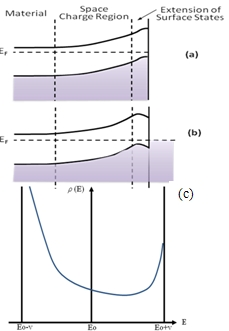Fig. 7:Heine's view of Fermi level pinning.  Surface-state band bending (a) on a free surface, (b) in the metal junction when φm < ĄŐSC. (c) Density of charge per unit energy in the semiconductor tails of the metal wave functions. Eo is the branch-point.

More rigorous self consistent pseudopotential calculations done by Louie et al.  showed that metal induced gap states were bulk like in metals and decayed rapidly into semiconductor with some of the characteristics of the semiconductor-vacuum surface states and not strongly dependent on the characteristics of each metal. With use of Thomas-Fermi screening a local density of only 0.02state/atom eV in the gap gives a screening length of about 3A, while Louie et al. show a density much greater than this for the first 6A at a metal-Si interface.

Tersoff's Model

Gap states can be expressed as a summation of valence and conduction bands. Charge neutrality requires occupation of MIGS which come primarily from the valence band, while leaving those of mainly conduction band character empty. Tersoff  proposed that Fermi level must fall at or near the energy where the gap states cross over from valence- to conduction band character. Just before the states spill over into the conduction band like states from valence band like states the MIGS DOS becomes minimum and the Fermi level is pinned precisely at this minimum. He found the branch point by using cell-averaged real space Green's function,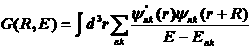where k is the Block wave vector, n is the band index, and ψ and E are the corresponding wave function and energy. R is suspiciously selected as (110).

The suggestion by Tersoff can be easily applied to estimate the band discontinuities of the semiconductor heterojunctions. Theoretical values obtained by taking the difference in EB in Table 1 are presented in table 2 and the resulting predictions are only slightly different from the experimental values in the small bandgap materials where the Fermi pinning are strong.

Summary

Metal and lightly doped semiconductor contact with different workfunction forms the Schottky barrier. The current flowing over the barrier represents thermionic emission behavior. Using capacitance-voltage measurement, the Schottky barrier height can be extracted. Theories to explain the Fermi level pinning of Schottky barrier were around for decades. Metal Induced Gap States model is supported by various experimental data and atomistic pseudopotential calculations.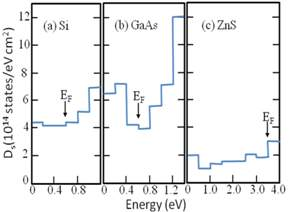Fig. 8:Surface State density calculated by Louise et al.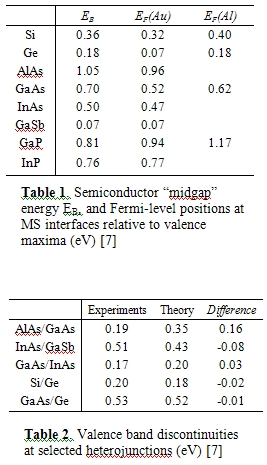© 2007 Donghyun Kim. The author grants permission to copy, distribute and display this work in unaltered form, with attribution to the author, for noncommercial purposes only. All other rights, including commercial rights, are reserved to the author.

References

 D. A. Neamen, Semiconductor physics & devices. 2nd Ed. (McGraw-Hill, 1997).

 A.M. Cowley et al., "Surface States and Barrier Height of Metal-Semiconductor Systems," J. Appl. Phys 36, 3212 (1965).

 J. Bardeen, "Surface States and Rectification at a Metal Semiconductor Contact," Phys. Rev. 71, 717 (1947).

 W.E. Spicer et al., "Unified Defect Model and Beyond," J. Vac. Sci. Tech. 17, 1019 (1980).

 V. Heine, "Theory of Surface States," Phys. Rev. 138, A1689 (1965).

 S. G. Louie et al., "Ionicity and the Theory of Schottky Barriers," Phys. Rev. B 15, 2154 (1977).

 J. Tersoff, "Schottky Barrier Heights and the Continuum of Gap States," Phys. Rev. Lett. 52, 465, (1984); ibid., "Theory of Semiconductor Heterojunctions: The role of Quantum Dipoles," Phys. Rev. B 30, 4874 (1984).

 S. Kurtin et al., "Fundamental Transition in the Electronic Nature of Solids," Phys. Rev. Lett. 22, 1433 (1969).

 Singh et al., "Reverse I-V and C-V Characteristics of Schottky Barrier Type Diodes on Zn Doped InP Epilayers Grown by Metalorganic Vapor Phase Epitaxy," J. Appl. Phys. 76, 2336 (1994).